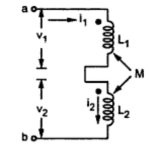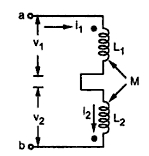### Inductive Coupling in Series

When two inductors having self inductances L1 and L2 are coupled in series, mutual inductance M exists between them. Two kinds of series connection are possible as follows :
1.1 Series Aiding
In this connection, two coils are connected in series such that their induced fluxes or voltages are additive in nature.Fig.  1
Here currents i1 and i2 is nothing but current i which is entering dots for both the coils.
Self induced voltage in coil 1 = v1 = -L1 (di/dt)
Self induced voltage in coil 2 = v = -L2 (di/dt)
Mutually induced voltage in coil 1 due to change in current in coil 2 = v1' = -M(di/dt)
Mutually induced voltage in coil 2 due to change in current in coil 1 = v2' = -M(di/dt)
Total induced voltage = v1 + v + v1' + v2'
= (L1 (di/dt) + L2 (di/dt) + M(di/dt) + M(di/dt))
= - (L1 + L2 + 2M)(di/dt)
If L is equivalent inductance across terminals a-b then total induced voltage in single inductance would be equal to -Leff  (di/dt). Comparing two voltages,
Leff  = L1 + L2 + 2M
1.1 Series Opposing
In this connection, two coils are connected in such a way that, the induced fluxes or voltages are of opposite polarities.Fig.  2
Here i1 and i2 is same series current ''i'' which is entering dot for coil L1 and leaving dot for coil L2.
Self induced voltage in coil 1 = -L1 (di/dt)
Self induced voltage in coil 2 = -L2 (di/dt)
Mutually induced voltage in coil 1 due to change in current in coil 2 =  v1'+ M (di/dt)
Also mutually induced voltage in coil 2 due to change in current in coil 1 = v2' +M(di/dt)
Therefore total induced voltage = v1+ v2 + v1' + v2'
= -L1 (di/dt) - L (di/dt) + M(di/dt) + (di/dt)
= -(L1 + L2 - 2M)(di/dt)
If L is equivalent inductance across terminals a and b then total induced voltage in single inductance would be equal to -Leff (di/dt)). Comparing two voltages,
Leff  = L1 + L2 - 2M